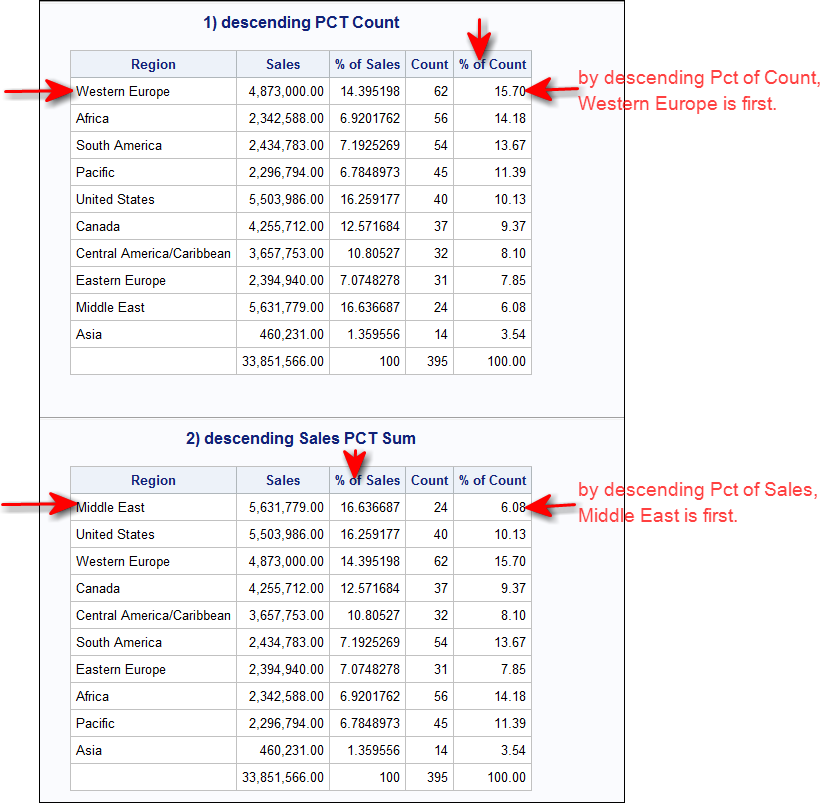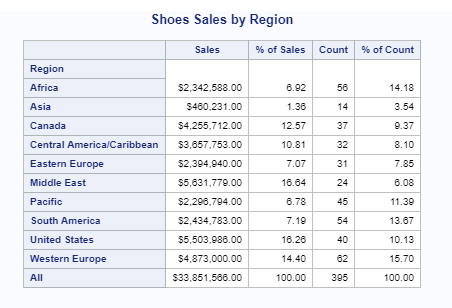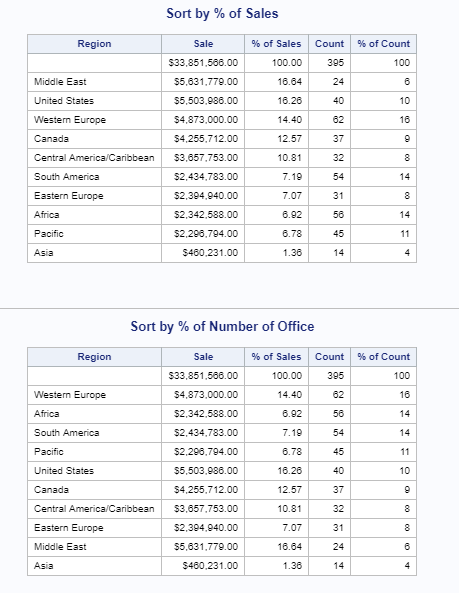## How to sort by colpctn of Proc Tabulate output?

Hi SAS Gurus,

I'd like to know if there is a way to sort the output of a Proc Tabulate by "colpctn" column. Here is an example.

Thank you so much.

Chin

1 ACCEPTED SOLUTION

Accepted Solutions

## Re: How to sort by colpctn of Proc Tabulate output?

Hi:

You cannot calculate a statistic and also sort by that statistic with either PROC TABULATE, or PROC REPORT.

I am confused by your region*all instead of region all (blank instead of *). Do you want a final total row at the bottom or not?

You are showing sales but asking for percent of N (count) for the column. Did you want to show the N on the report? Do you want the report sorted by the descending percent of the count or by the descending percent of SALES??? It makes a difference as to the final order, as shown below:For either of the above outputs, you have to make 2 passes. 1) summarize and calc percents in PROC TABULATE and let TABULATE create an output dataset, then 2) use PROC SORT to do the descending sort. Finally, use either PROC TABULATE or PROC REPORT to display the final report.

Cynthia

4 REPLIES 4

## Re: How to sort by colpctn of Proc Tabulate output?

Hi:

You cannot calculate a statistic and also sort by that statistic with either PROC TABULATE, or PROC REPORT.

I am confused by your region*all instead of region all (blank instead of *). Do you want a final total row at the bottom or not?

You are showing sales but asking for percent of N (count) for the column. Did you want to show the N on the report? Do you want the report sorted by the descending percent of the count or by the descending percent of SALES??? It makes a difference as to the final order, as shown below:For either of the above outputs, you have to make 2 passes. 1) summarize and calc percents in PROC TABULATE and let TABULATE create an output dataset, then 2) use PROC SORT to do the descending sort. Finally, use either PROC TABULATE or PROC REPORT to display the final report.

Cynthia

## Re: How to sort by colpctn of Proc Tabulate output?

Hi Cynthia,

Thank you very much for your well documented solution image. You have clarified once and for all that one can't sort a statistic output in TABULATE in one step. Your questions had me realized that colpctsum instead of colpctn is what I should use. Initially I didn't know about colpctsum. Based on your solution I wrote the following code. Please comment.

```proc tabulate data=sashelp.shoes out=shoes_by_reg;
class region;
var sales;
table region all, sales=""*(sum="Sales" * f=dollar14.2 colpctsum='% of Sales '*f=12.2) n="Count" colpctn="% of Count"*f=12.2;
run;

proc sort data=shoes_by_reg out=shoes_by_sales (drop=_Type_ _page_ _table_);
by descending sales_PctSum_0_sales;
label Sales_Sum='Sale' Sales_PctSum_0_sales='  % of Sales' N='Count' PctN_0='  % of Count';
format sales_sum dollar14.2;
format Sales_PctSum_0_sales 6.2;
run;

proc report data=shoes_by_sales;
title "Sort by % of Sales";
columns region sales_sum sales_pctsum_0_sales n Pctn_0;
define region/display;
define sales_sum/display;
define sales_pctsum_0_sales/display;
define n/display;
define Pctn_0/display f=5.;
run;

proc sort data=shoes_by_reg out=shoes_by_n (drop=_Type_ _page_ _table_);
by descending PctN_0;
label Sales_Sum='Sale' Sales_PctSum_0_sales='  % of Sales' N='Count' PctN_0='  % of Count';
format sales_sum dollar14.2;
format Sales_Pctsum_0_sales 6.2;
run;

proc report data=shoes_by_n;
title "Sort by % of Number of Office";
columns region sales_sum sales_pctsum_0_sales n Pctn_0;
define region/display;
define sales_sum/display;
define sales_pctsum_0_sales/display;
define n/display;
define Pctn_0/display f=5.;
run;```

The output mimic yours,The output dataset "shoes_by_reg" omitted "ALL" for total sum under the "Region" column. So all subsequent procedures do not have "ALL". Would you have a suggestion to "fill in" this ALL in the dataset "shoes_by_reg"?

Lastly you mentioned that to use Proc Tabulate as a 2nd pass to re-tabulate the sorted datasets. Would you please provide a solution to demonstrate how to do that. I struggled with it and couldn't to have Tabulate to simply list columns as I did with Proc Report.

Thank you very much for the expert help.

Chin

## Re: How to sort by colpctn of Proc Tabulate output?

Hi:

The top line of your output shows the Overall Total -- this is because TABULATE puts the "grand total" as the value of _TYPE_ set to 0. In my example code below, I only get the _TYPE_ values = 1 so I can put the All or Grand Total at the bottom myself. Here's the code I used. Example #3 is the TABULATE method. I prefer the PROC REPORT method #2.

Cynthia

``````** calc percents with TABULATE;
proc tabulate data=sashelp.shoes
PctN_0=PCTCount ));
title 'Start: First Summarize and calc Percents';
class region;
var sales;
table region all='Total',
sales=''*(sum*f=dollar14.2 colpctsum*f=9.2) n colpctn*f=12.2 ;
run;
title;

** now sort the output data work.tabout;
by descending PctCount;
where _type_ = '1';
run;

proc report data=work.sortout1;
title '1) PROC REPORT descending PCT Count';
column region sales salesPctSum n PctCount;
define sales/ sum 'Sales' f=comma14.2;
define salesPctSum / sum '% of Sales' f=6.2;
define n / sum 'Count' f=comma12.;
define pctCount/ '% of Count' sum f=6.2;
compute after;
region = 'Total';
endcomp;
run;
title;

by descending SalesPctSum;
where _type_ = '1';
run;

proc report data=work.sortout2;
title '2) PROC REPORT descending Sales PCT Sum';
column region sales salesPctSum n PctCount;
define sales/ sum 'Sales' f=comma14.2;
define salesPctSum / sum '% of Sales' f=6.2;
define n / sum 'Count' f=comma12.;
define pctCount/ '% of Count' sum f=6.2;
compute after;
region = 'Total';
endcomp;
run;
title;

by descending SalesPctSum;
where _type_ = '1';
run;

proc tabulate data=work.sortout3;
title '3) PROC TABULATE descending Sales PCT Sum';
class region / order=data;
var sales salespctsum n pctcount;
sales*f=comma14.2 salespctsum*f=6.2 n*f=comma12. pctcount*f=6.2
/ style_precedence=row;
keylabel sum=' '
all='Total';
run;
title;

``````

## Re: How to sort by colpctn of Proc Tabulate output?

Hi Cynthia,

Thank you very much for spending the time to provide the brilliant code. I will continue to digest some of the great techniques embedded. Talk to you next time.

Chin

Discussion stats
• 4 replies
• 2941 views
• 0 likes
• 2 in conversation